# Independent Samples T-Test - Equal Variance

Where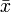$\bar{x}$ and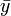$\bar{y}$ are the two means and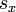$s_x$ and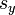$s_y$ respectively are their Standard Deviations, and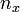$n_x$ and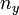$n_y$ are their sample sizes respectively, the test statistic is: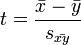$t=\frac{\bar{x}-\bar{y}}{s_\bar{xy}}$,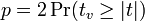$p = 2\Pr(t_v \ge |t|)$,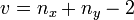$v = n_x + n_y - 2$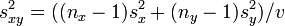$s^2_{xy} = ((n_x - 1) s^2_x + (n_y - 1) s^2_y) / v$, and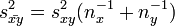$s^2_\bar{xy} = s^2_{xy}(n^{-1}_x + n^{-1}_y)$.